# A Ratio That Glitters Exploring Golden Ratios Golden

• Slides: 18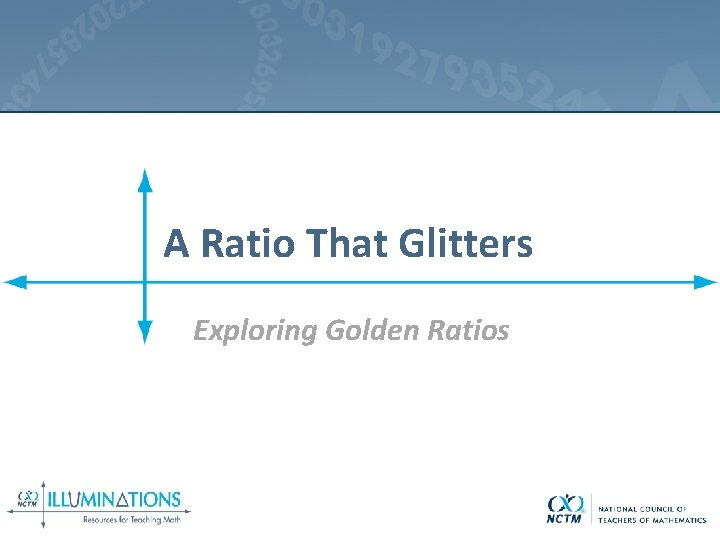A Ratio That Glitters Exploring Golden RatiosGolden Ratio in Architecture The Pyramid of Khufu has the Golden Ratio in the ratio of the height of the pyramid with the length of half the base of the pyramid.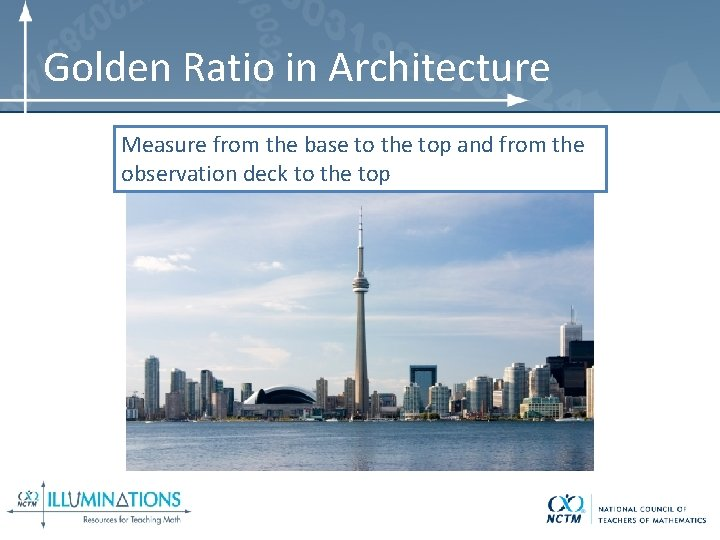Golden Ratio in Architecture Measure from the base to the top and from the observation deck to the top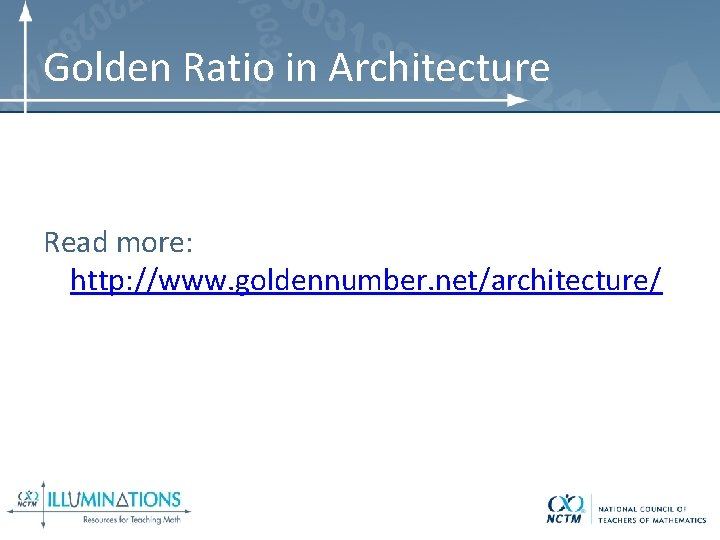Golden Ratio in Architecture Read more: http: //www. goldennumber. net/architecture/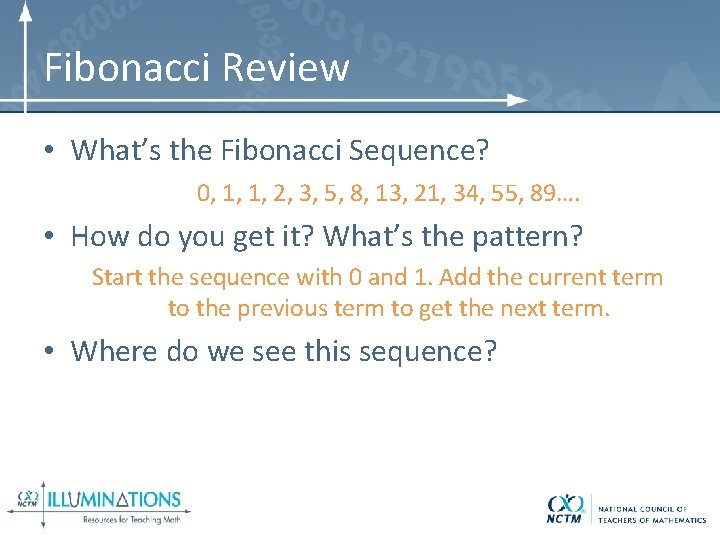Fibonacci Review • What’s the Fibonacci Sequence? 0, 1, 1, 2, 3, 5, 8, 13, 21, 34, 55, 89…. • How do you get it? What’s the pattern? Start the sequence with 0 and 1. Add the current term to the previous term to get the next term. • Where do we see this sequence?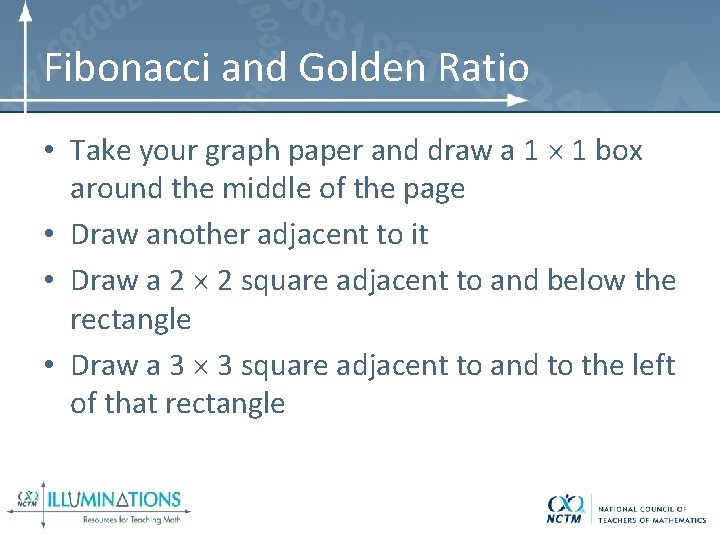Fibonacci and Golden Ratio • Take your graph paper and draw a 1 1 box around the middle of the page • Draw another adjacent to it • Draw a 2 2 square adjacent to and below the rectangle • Draw a 3 3 square adjacent to and to the left of that rectangle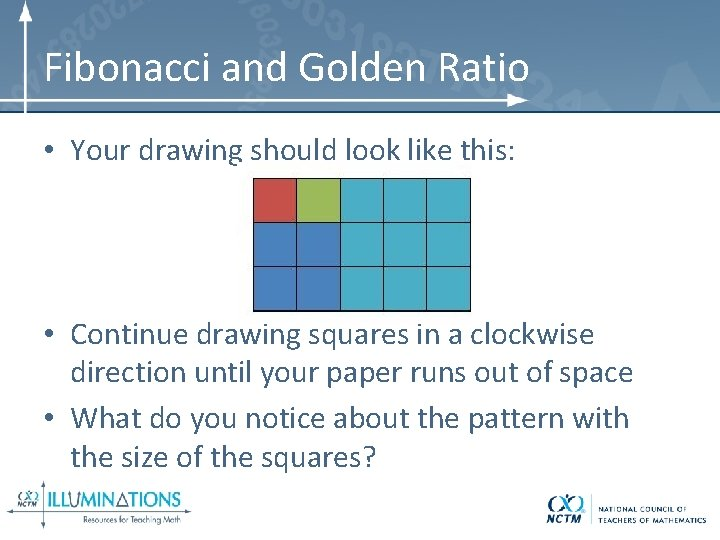Fibonacci and Golden Ratio • Your drawing should look like this: • Continue drawing squares in a clockwise direction until your paper runs out of space • What do you notice about the pattern with the size of the squares?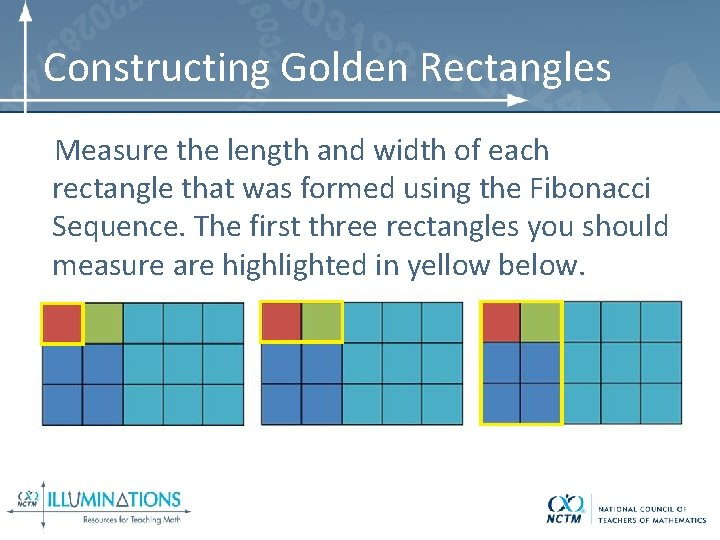Constructing Golden Rectangles Measure the length and width of each rectangle that was formed using the Fibonacci Sequence. The first three rectangles you should measure are highlighted in yellow below.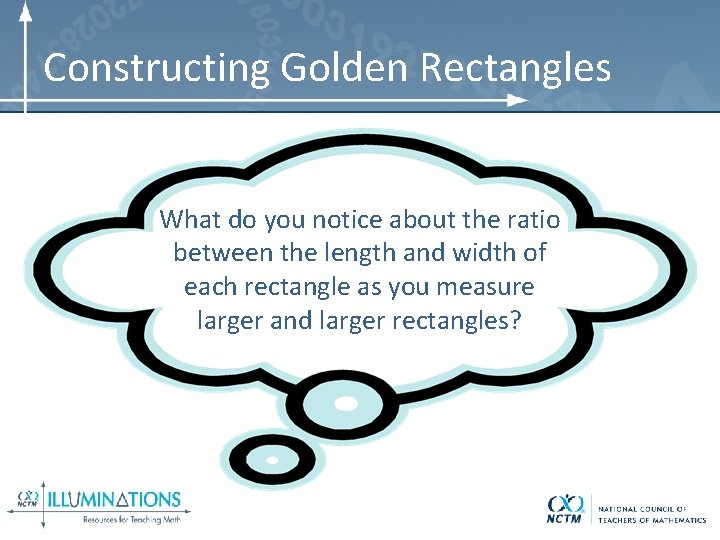Constructing Golden Rectangles What do you notice about the ratio between the length and width of each rectangle as you measure larger and larger rectangles?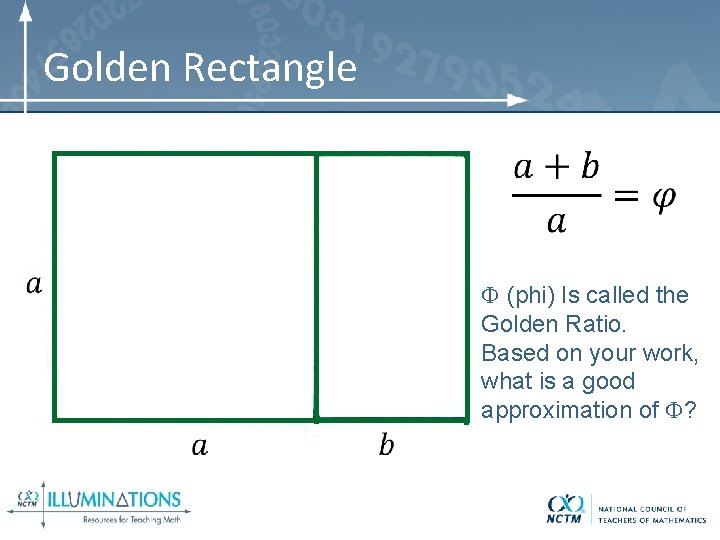Golden Rectangle (phi) Is called the Golden Ratio. Based on your work, what is a good approximation of ?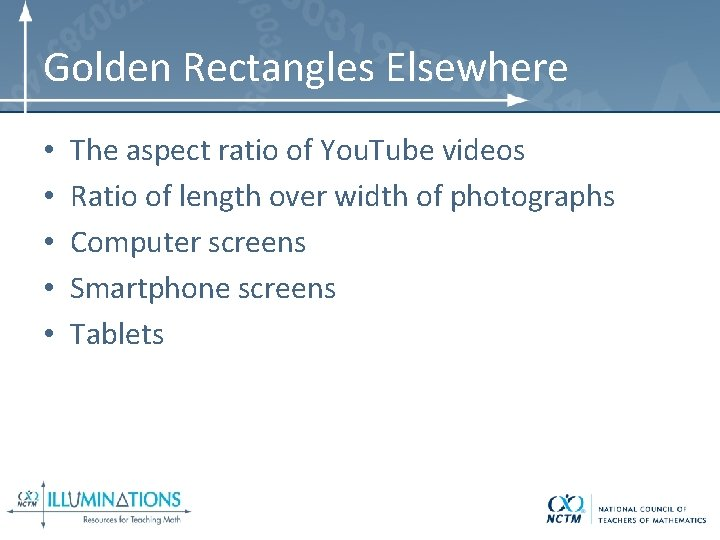Golden Rectangles Elsewhere • • • The aspect ratio of You. Tube videos Ratio of length over width of photographs Computer screens Smartphone screens TabletsConstructing Golden Rectangles • Draw a square • Find the midpoint of the side of the square (A)Constructing Golden Rectangles • Extend one side • Draw an arc of radius AB, centered at the midpoint, and have it intersect the extended side of the square (C).Constructing Golden Rectangles • Construct a perpendicular line at the intersection of the arc and the side.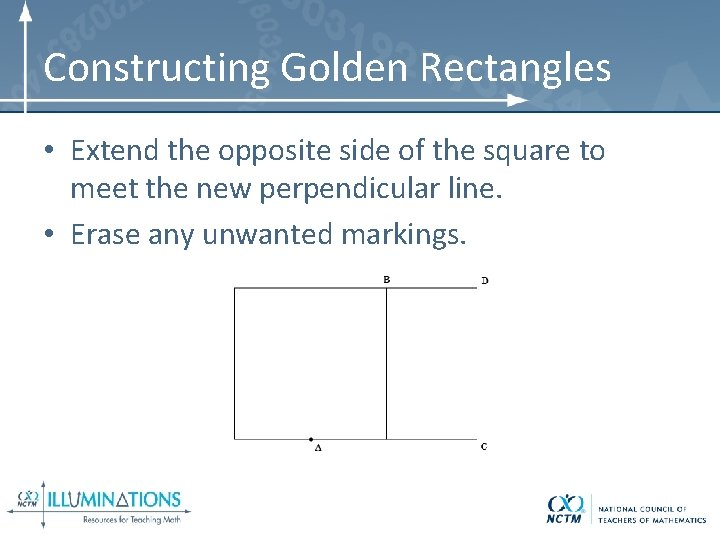Constructing Golden Rectangles • Extend the opposite side of the square to meet the new perpendicular line. • Erase any unwanted markings.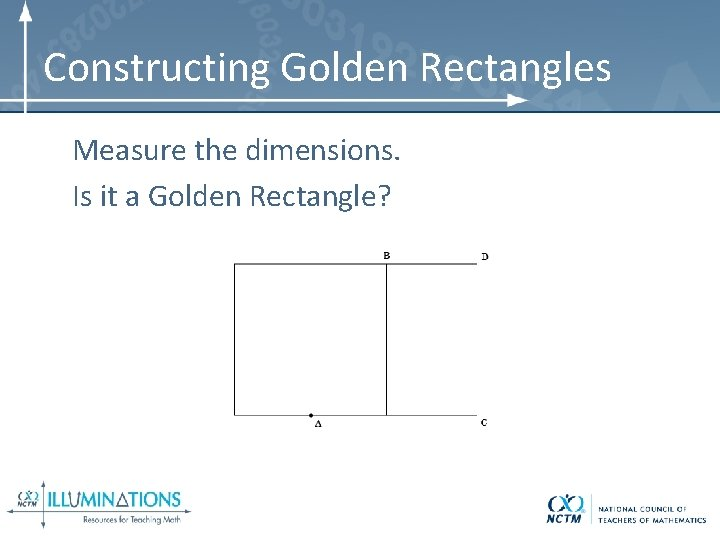Constructing Golden Rectangles Measure the dimensions. Is it a Golden Rectangle?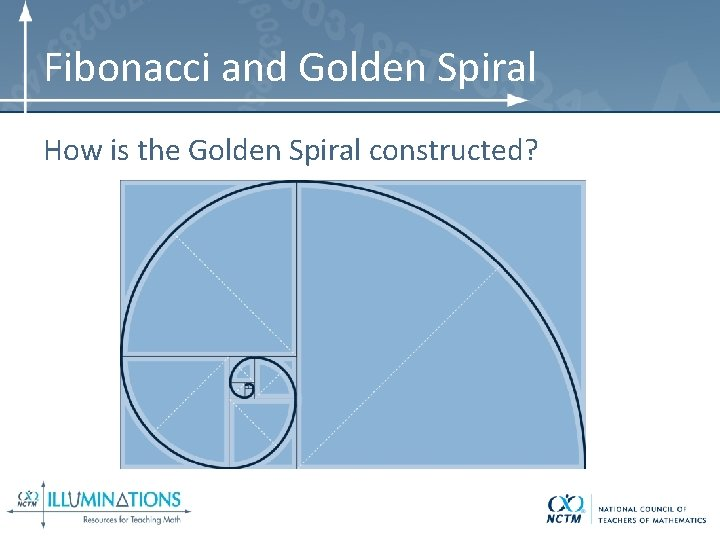Fibonacci and Golden Spiral How is the Golden Spiral constructed?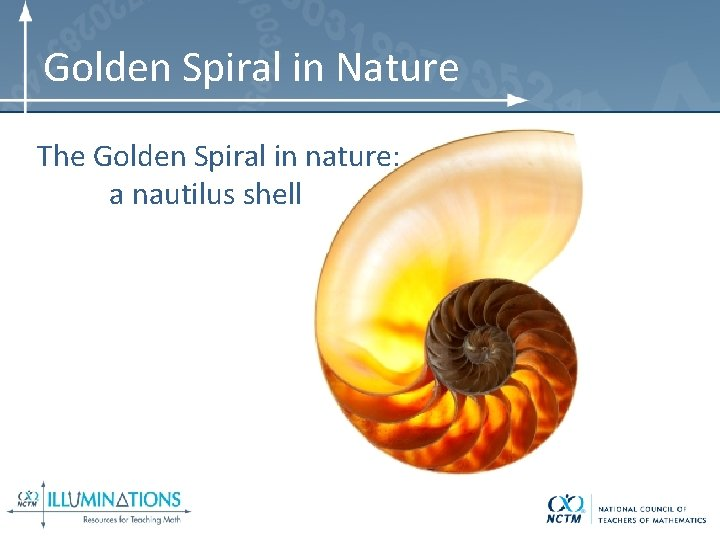Golden Spiral in Nature The Golden Spiral in nature: a nautilus shell